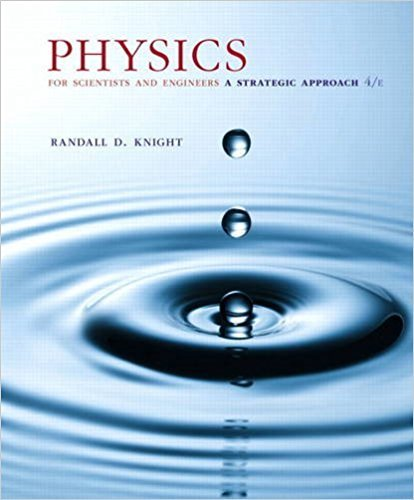×

# a. Calculate and graph the hydrogen radial wave functionISBN: 9780134081496 191

## Solution for problem 41.67 Chapter 41

Physics for Scientists and Engineers: A Strategic Approach, Standard Edition (Chs 1-36) | 4th Edition

• Textbook Solutions
• 2901 Step-by-step solutions solved by professors and subject experts
• Get 24/7 help from StudySoup virtual teaching assistantsPhysics for Scientists and Engineers: A Strategic Approach, Standard Edition (Chs 1-36) | 4th Edition

4 5 0 301 Reviews
16
1
Problem 41.67

a. Calculate and graph the hydrogen radial wave function R2p1r2 over the interval 0 r 8aB. b. Determine the value of r (in terms of aB) for which R2p1r2 is a maximum. c. Example 41.3 and Figure 41.7 showed that the radial probability density for the 2p state is a maximum at r = 4aB. Explain why this differs from your answer to part b

Step-by-Step Solution:
Step 1 of 3

ilami (ki2628) – Homework #2 – reichl – (55945) 1 This print-out should have 18 questions. Multiple-choice questions may continue on 003 (part 3 of 3) 10.0 points the next column or page – ﬁnd all choices What is the position...

Step 2 of 3

Step 3 of 3

##### ISBN: 9780134081496

Physics for Scientists and Engineers: A Strategic Approach, Standard Edition (Chs 1-36) was written by and is associated to the ISBN: 9780134081496. This textbook survival guide was created for the textbook: Physics for Scientists and Engineers: A Strategic Approach, Standard Edition (Chs 1-36), edition: 4. The answer to “a. Calculate and graph the hydrogen radial wave function R2p1r2 over the interval 0 r 8aB. b. Determine the value of r (in terms of aB) for which R2p1r2 is a maximum. c. Example 41.3 and Figure 41.7 showed that the radial probability density for the 2p state is a maximum at r = 4aB. Explain why this differs from your answer to part b” is broken down into a number of easy to follow steps, and 65 words. This full solution covers the following key subjects: . This expansive textbook survival guide covers 42 chapters, and 4463 solutions. Since the solution to 41.67 from 41 chapter was answered, more than 226 students have viewed the full step-by-step answer. The full step-by-step solution to problem: 41.67 from chapter: 41 was answered by , our top Physics solution expert on 12/28/17, 08:06PM.

Unlock Textbook Solution

a. Calculate and graph the hydrogen radial wave function

×